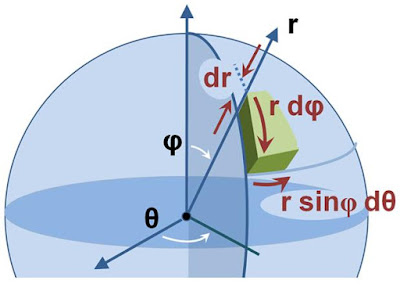# Derivation of Volume of Sphere

We can derive the formula for volume of sphere in a number of ways. The most fundamental method to find out volume of any three dimensional symmetrical structure comprises of the following methodology ౼ first pick any arbitrary infinitesimal volumetric element inside the given structure, write the expression for its small volume (in terms of its position and infinitesimal dimensions) and then simply integrate this expression for the entire structure utilizing calculus. The same approach we are going to use to derive the volume expression of a sphere. Here one important thing to note is that the elementry volume can be chosen in a variety of ways (depending upon the coordinate systems) but finally, on integrating under proper limits, we get the same result V = (4/3)πr³.

### METHOD 1

A spherical volume can be assumed to be constituted of a large number of thin circular disks of continuously varying radii kept over one another with their centres collinear. We have to choose one of these elementary disks. See the drawing given below. A thin disk element of radius r and thickness dy located at a distance of y from the horizontal axis has been focused. Its volume can be written as the multiplication of base area (or simply area) πr² and thickness dy. Further, the disc radius r can be expressed in terms of vertical dimension y using the Pythagoras theorem.

### METHOD 2

We can also choose a thin spherical shell element of radius r (such that 0 < r < R) and infinitesimal thickness dr. The summation of volumes occupied by all such elements present from radius 0 to R will yield the total volume.

### METHOD 3

The formula of volume can also be derived by working in spherical system of coordinates. We choose a differential volume element in terms of r, Φ and Ɵ
where r is the distance of the volume element from origin, Φ is the angle made by the line joining the volumetric element to the center with the vertical axis and Ɵ the azimuthal angle as depicted in the figure below.Image Source : https://commons.m.wikimedia.org/wiki

Approximating the volumetric element as a cuboid, the differential volume can be written as

### METHOD 4

One way for imagining a spherical volume can also be to approx it as being constitued of large number of infinitesimal cones (all identical), each one having its vertex located at the centre of the sphere and their small bases lying along the outer surface of the sphere. Obviously the height of these cones will be same as the radius of the sphere and their base radii will be infinitesimally small say dr. Now to obtain the total volume of the sphere, first write the expression for the small volume of an elementary cone and then integrate it.

Hindi Hearts